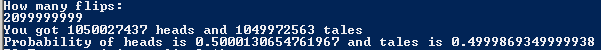# FINAL #1

## Code

```//colin hinton
//5th period
//Coin.java
//1/20/2015

import java.util.Scanner;
import java.util.Random;

public class Coin
{
public static void main (String [] args)
{
Scanner kb = new Scanner(System.in);
Random r = new Random();

int tale = 0; //the count for heads and tales after the flipping begins
System.out.println("How many flips: ");
int flip = kb.nextInt();
if (flip > 0 && flip < 2100000000) //insures that flips are not too little or large
{
for (int x = 0; x <= flip; x++)
{
int y = 1 + r.nextInt(2); // determins which pile the rng goes to based on either 1 or 2
if(y == 1)
{
}
else if (y == 2)
{
tale++;
}
}
System.out.println("You got " + head + " heads and " + tale + " tales");
double probH = (double)head / flip;
double probT = (double)tale / flip;
System.out.println("Probability of heads is " + probH + " and tales is " + probT);
}
else
{
System.out.println("YOU DONT DESERVE MY PROGRAM"); //bad yokes
}
}
}
//The larger the number the more likley you will achieve a true 50/50 chance
//So for this program that number would be 2099999999

```

### Picture of the output